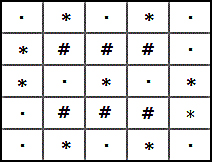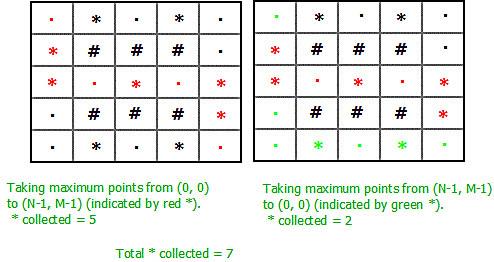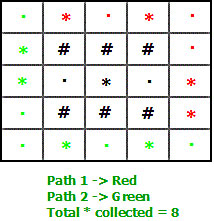# Maximum points from top left of matrix to bottom right and return back

Given a matrix of size N X M consisting of ‘#’, ‘.’ and ‘*’. ‘#’ means blocked path, ‘.’ means walkable path and ‘*’ means points that you have to collect. Now consider you are at the top left of the matrix. You have to reach the bottom right of the matrix and come back to the top left. When you are moving top left to bottom right, you are allowed to walk right or down. And when you are moving the bottom right to top left, you are allowed to walk left or up. The task is to find the maximum points you can grab during your whole journey. Points once taken will be converted into ‘.’ i.e walkable path.

Examples:

```Input : N = 3, M = 4
****
.##.
.##.
****
Output : 8

Input : N = 9, M = 7
*........
.....**#.
..**...#*
..####*#.
.*.#*.*#.
...#**...
*........
Output : 7
```

## Recommended: Please try your approach on {IDE} first, before moving on to the solution.

If you consider two paths – one from (0, 0) to (N – 1, M – 1) and another from (N – 1, M – 1) to (0, 0) and collect maximum * in each path. You might end up with wrong answer. If you add the answer of both the paths independently, you will be calculating the intersecting points once again while backtracking. Essentially, ending up with the same path. Even if you keep a visited array, marking every * on the first path, you will still not end up with the correct answer.
Consider the following example:If we consider as two independent path, thentotal * collected is 7.
Whereas the maximum points collected can be 8 by following paths.There are 4 * on each path. Hence, total = 8.
Thus we see that the optimal solution is not the sum of optimal solutions of both the path independently. The optimal answer for one does not ensure an optimal answer.

So, we will have to calculate both the paths simultaneously. This is needed because the answer for path 2 depends on the route chosen by path 1. Simultaneous calculations can be done by considering two paths from (0, 0) to (N-1, M-1) and making four decisions at each position (two for each).
So instead of two traveling one path from top left to the bottom right and other from the bottom right to top left, we will travel two paths from (0, 0) to (N-1, M-1) simultaneously, so at each step, we take one step for both paths. So our state will consist of (x1, y1, x2, y2) where (x1, y1) is the position of the first path and (x2, y2) is the position of the second tourist in the grid.
Points to notice:
1. At each step either step can move right or down, so we have 4 choices for movement(2 choices for each path).
2. If both paths are on the same cell (x1 == x2 and y1 == y2) then we can add only 1 to result if that cell has *.
3. We can reduce the complexity by reducing the state dimension from 4 to 3. If we know the position of first path (x1, y1) the x coordinate of second path x2, then we must have x1 + y1 = x2 + y2 since both path cover the same distance in the same amount of time. So y2 = x1 + y1 – x2 and our state depends only on (x1, y1, x2).

Below is the implementation of this approach:

## C++

 `// CPP program to find maximum points that can  ` `// be collected in a journey from top to bottom  ` `// and then back from bottom to top, ` `#include ` `#define MAX 5 ` `#define N 5 ` `#define M 5 ` `#define inf 100000 ` `using` `namespace` `std; ` ` `  `// Calculating the points at a (row1, col1) &&  ` `// (row2, col2) from path1 && path2 ` `int` `cost(``char` `grid[][M], ``int` `row1, ``int` `col1,  ` `                           ``int` `row2, ``int` `col2) ` `{ ` `    ``// If both path is at same cell ` `    ``if` `(row1 == row2 && col1 == col2) { ` ` `  `        ``// If the cell contain *, return 1 ` `        ``if` `(grid[row1][col1] == ``'*'``) ` `            ``return` `1; ` ` `  `        ``// else return 0. ` `        ``return` `0; ` `    ``} ` ` `  `    ``int` `ans = 0; ` ` `  `    ``// If path 1 contain *, add to answer. ` `    ``if` `(grid[row1][col1] == ``'*'``) ` `        ``ans++; ` ` `  `    ``// If path  contain *, add to answer. ` `    ``if` `(grid[row2][col2] == ``'*'``) ` `        ``ans++; ` ` `  `    ``return` `ans; ` `} ` ` `  `// Calculate the maximum points that can be ` `// collected. ` `int` `solve(``int` `n, ``int` `m, ``char` `grid[][M], ` `         ``int` `dp[MAX][MAX][MAX], ``int` `row1,  ` `                      ``int` `col1, ``int` `row2) ` `{ ` `    ``int` `col2 = (row1 + col1) - (row2); ` ` `  `    ``// If both path reach the bottom right cell ` `    ``if` `(row1 == n - 1 && col1 == m - 1 && ` `        ``row2 == n - 1 && col2 == m - 1) ` `        ``return` `0; ` ` `  `    ``// If moving out of grid ` `    ``if` `(row1 >= n || col1 >= m ||  ` `        ``row2 >= n || col2 >= m) ` `        ``return` `-1 * inf; ` ` `  `    ``// If already calculated, return the value ` `    ``if` `(dp[row1][col1][row2] != -1) ` `        ``return` `dp[row1][col1][row2]; ` ` `  `    ``// Variable for 4 options. ` `    ``int` `ch1 = -1 * inf, ch2 = -1 * inf; ` `    ``int` `ch3 = -1 * inf, ch4 = -1 * inf; ` ` `  `    ``// If path 1 is moving right and path 2  ` `    ``// is moving down. ` `    ``if` `(grid[row1][col1 + 1] != ``'#'` `&&  ` `        ``grid[row2 + 1][col2] != ``'#'``) ` `      ``ch1 = cost(grid, row1, col1 + 1, row2 + 1, col2) +  ` `        ``solve(n, m, grid, dp, row1, col1 + 1, row2 + 1); ` ` `  `    ``// If path 1 is moving right and path 2 is moving ` `    ``// right. ` `    ``if` `(grid[row1][col1 + 1] != ``'#'` `&&  ` `        ``grid[row2][col2 + 1] != ``'#'``) ` `      ``ch2 = cost(grid, row1, col1 + 1, row2, col2 + 1) +  ` `            ``solve(n, m, grid, dp, row1, col1 + 1, row2); ` ` `  `    ``// If path 1 is moving down and path 2 is moving right. ` `    ``if` `(grid[row1 + 1][col1] != ``'#'` `&&  ` `        ``grid[row2][col2 + 1] != ``'#'``) ` `     ``ch3 = cost(grid, row1 + 1, col1, row2, col2 + 1) +  ` `           ``solve(n, m, grid, dp, row1 + 1, col1, row2); ` ` `  `    ``// If path 1 is moving down and path 2 is moving down. ` `    ``if` `(grid[row1 + 1][col1] != ``'#'` `&&  ` `        ``grid[row2 + 1][col2] != ``'#'``) ` `      ``ch4 = cost(grid, row1 + 1, col1, row2 + 1, col2) + ` `         ``solve(n, m, grid, dp, row1 + 1, col1, row2 + 1); ` ` `  `    ``// Returning the maximum of 4 options. ` `    ``return` `dp[row1][col1][row2] = max({ch1, ch2, ch3, ch4}); ` `} ` ` `  `// Wrapper Function ` `int` `wrapper(``int` `n, ``int` `m, ``char` `grid[N][M]) ` `{ ` `    ``int` `ans = 0; ` `    ``int` `dp[MAX][MAX][MAX]; ` `    ``memset``(dp, -1, ``sizeof` `dp); ` ` `  `    ``// If last bottom right cell is blcoked ` `    ``if` `(grid[n - 1][m - 1] == ``'#'` `|| grid == ``'#'``) ` `        ``ans = -1 * inf; ` ` `  `    ``// If top left cell contain * ` `    ``if` `(grid == ``'*'``) ` `        ``ans++; ` `    ``grid = ``'.'``; ` ` `  `    ``// If bottom right cell contain * ` `    ``if` `(grid[n - 1][m - 1] == ``'*'``) ` `        ``ans++; ` `    ``grid[n - 1][m - 1] = ``'.'``; ` ` `  `    ``ans += solve(n, m, grid, dp, 0, 0, 0); ` `    ``return` `max(ans, 0); ` `} ` ` `  `// Driven Program ` `int` `main() ` `{ ` `    ``int` `n = 5, m = 5; ` ` `  `    ``char` `grid[N][M] = { ` `        ``{ ``'.'``, ``'*'``, ``'.'``, ``'*'``, ``'.'` `}, ` `        ``{ ``'*'``, ``'#'``, ``'#'``, ``'#'``, ``'.'` `}, ` `        ``{ ``'*'``, ``'.'``, ``'*'``, ``'.'``, ``'*'` `}, ` `        ``{ ``'.'``, ``'#'``, ``'#'``, ``'#'``, ``'*'` `}, ` `        ``{ ``'.'``, ``'*'``, ``'.'``, ``'*'``, ``'.'` `} ` `    ``}; ` ` `  `    ``cout << wrapper(n, m, grid) << endl; ` `    ``return` `0; ` `} `

## Python3

 `# Python3 program to find maximum points  ` `# that can be collected in a journey from  ` `# top to bottom and then back from bottom to top,  ` `MAX` `=` `5` `N ``=` `5` `M ``=` `5` `inf ``=` `100000` ` `  `# Calculating the points at a (row1, col1) and  ` `# (row2, col2) from path1 and path2  ` `def` `cost(grid, row1, col1, row2, col2):  ` ` `  `    ``# If both path is at same cell  ` `    ``if` `(row1 ``=``=` `row2 ``and` `col1 ``=``=` `col2):  ` ` `  `        ``# If the cell contain *, return 1  ` `        ``if` `(grid[row1][col1] ``=``=` `'*'``):  ` `            ``return` `1` ` `  `        ``# else return 0.  ` `        ``return` `0` ` `  `    ``ans ``=` `0` ` `  `    ``# If path 1 contain *, add to answer.  ` `    ``if` `(grid[row1][col1] ``=``=` `'*'``):  ` `        ``ans ``+``=` `1` ` `  `    ``# If path contain *, add to answer.  ` `    ``if` `(grid[row2][col2] ``=``=` `'*'``):  ` `        ``ans ``+``=` `1` ` `  `    ``return` `ans  ` ` `  `# Calculate the maximum points that can be  ` `# collected.  ` `def` `solve(n, m, grid, dp, row1, col1, row2):  ` ` `  `    ``col2 ``=` `(row1 ``+` `col1) ``-` `(row2)  ` ` `  `    ``# If both path reach the bottom right cell  ` `    ``if` `(row1 ``=``=` `n ``-` `1` `and` `col1 ``=``=` `m ``-` `1` `and` `        ``row2 ``=``=` `n ``-` `1` `and` `col2 ``=``=` `m ``-` `1``):  ` `        ``return` `0` ` `  `    ``# If moving out of grid  ` `    ``if` `(row1 >``=` `n ``or` `col1 >``=` `m ``or`  `        ``row2 >``=` `n ``or` `col2 >``=` `m):  ` `        ``return` `-``1` `*` `inf  ` ` `  `    ``# If already calculated, return the value  ` `    ``if` `(dp[row1][col1][row2] !``=` `-``1``):  ` `        ``return` `dp[row1][col1][row2]  ` ` `  `    ``# Variable for 4 options.  ` `    ``ch1 ``=` `-``1` `*` `inf ` `    ``ch2 ``=` `-``1` `*` `inf  ` `    ``ch3 ``=` `-``1` `*` `inf ` `    ``ch4 ``=` `-``1` `*` `inf  ` ` `  `    ``# If path 1 is moving right and path 2  ` `    ``# is moving down.  ` `    ``if` `(col1 ``+` `1` `< m ``and` `row2 ``+` `1` `< n ``and`  `          ``grid[row1][col1 ``+` `1``] !``=` `'#'` `and`  `          ``grid[row2 ``+` `1``][col2] !``=` `'#'``):  ` `        ``ch1 ``=` `cost(grid, row1, col1 ``+` `1``, row2 ``+` `1``, col2) ``+` `\ ` `             ``solve(n, m, grid, dp, row1, col1 ``+` `1``, row2 ``+` `1``)  ` ` `  `    ``# If path 1 is moving right and path 2  ` `    ``# is moving right.  ` `    ``if` `(col1 ``+` `1` `< m ``and` `col2 ``+` `1` `< m ``and`  `          ``grid[row1][col1 ``+` `1``] !``=` `'#'` `and`  `          ``grid[row2][col2 ``+` `1``] !``=` `'#'``):  ` `        ``ch2 ``=` `cost(grid, row1, col1 ``+` `1``, row2, col2 ``+` `1``) ``+` `\ ` `             ``solve(n, m, grid, dp, row1, col1 ``+` `1``, row2)  ` ` `  `    ``# If path 1 is moving down and path 2 ` `    ``# is moving right.  ` `    ``if` `(row1 ``+` `1` `< n ``and` `col2 ``+` `1` `< m ``and`  `          ``grid[row1 ``+` `1``][col1] !``=` `'#'` `and`  `          ``grid[row2][col2 ``+` `1``] !``=` `'#'``):  ` `        ``ch3 ``=` `cost(grid, row1 ``+` `1``, col1, row2, col2 ``+` `1``) ``+` `\ ` `             ``solve(n, m, grid, dp, row1 ``+` `1``, col1, row2)  ` ` `  `    ``# If path 1 is moving down and path 2 is moving down.  ` `    ``if` `(row1 ``+` `1` `< n ``and` `row2 ``+` `1` `< n ``and`  `          ``grid[row1 ``+` `1``][col1] !``=` `'#'` `and`  `          ``grid[row2 ``+` `1``][col2] !``=` `'#'``):  ` `        ``ch4 ``=` `cost(grid, row1 ``+` `1``, col1, row2 ``+` `1``, col2) ``+` `\ ` `             ``solve(n, m, grid, dp, row1 ``+` `1``, col1, row2 ``+` `1``)  ` ` `  `    ``# Returning the maximum of 4 options.  ` `    ``dp[row1][col1][row2] ``=` `max``(ch1, ch2, ch3, ch4) ` `    ``return` `dp[row1][col1][row2] ` ` `  `# Wrapper Function  ` `def` `wrapper(n, m, grid):  ` ` `  `    ``ans ``=` `0` ` `  `    ``dp ``=` `[[[``-``1``] ``*` `MAX` `for` `i ``in` `range``(``MAX``)]  ` `                      ``for` `j ``in` `range``(``MAX``)]  ` ` `  `    ``# If last bottom right cell is blcoked  ` `    ``if` `(grid[n ``-` `1``][m ``-` `1``] ``=``=` `'#'` `or`  `        ``grid[``0``][``0``] ``=``=` `'#'``):  ` `        ``ans ``=` `-``1` `*` `inf  ` ` `  `    ``# If top left cell contain *  ` `    ``if` `(grid[``0``][``0``] ``=``=` `'*'``):  ` `        ``ans ``+``=` `1` `    ``grid[``0``][``0``] ``=` `'.'` ` `  `    ``# If bottom right cell contain *  ` `    ``if` `(grid[n ``-` `1``][m ``-` `1``] ``=``=` `'*'``):  ` `        ``ans ``+``=` `1` `    ``grid[n ``-` `1``][m ``-` `1``] ``=` `'.'` ` `  `    ``ans ``+``=` `solve(n, m, grid, dp, ``0``, ``0``, ``0``)  ` `    ``return` `max``(ans, ``0``)  ` ` `  `# Driver Code ` `if` `__name__ ``=``=` `'__main__'``:  ` `    ``n ``=` `5` `    ``m ``=` `5` ` `  `    ``grid ``=` `[[ ``'.'``, ``'*'``, ``'.'``, ``'*'``, ``'.'` `],  ` `            ``[ ``'*'``, ``'#'``, ``'#'``, ``'#'``, ``'.'` `],  ` `            ``[ ``'*'``, ``'.'``, ``'*'``, ``'.'``, ``'*'` `],  ` `            ``[ ``'.'``, ``'#'``, ``'#'``, ``'#'``, ``'*'` `],  ` `            ``[ ``'.'``, ``'*'``, ``'.'``, ``'*'``, ``'.'` `]]  ` `     `  `    ``print``(wrapper(n, m, grid))  ` `     `  `# This code is contributed by ashutosh450 `

Output:

```8
```

Time Complexity: O(N^3)

This article is contributed by Anuj Chauhan. If you like GeeksforGeeks and would like to contribute, you can also write an article using contribute.geeksforgeeks.org or mail your article to contribute@geeksforgeeks.org. See your article appearing on the GeeksforGeeks main page and help other Geeks.